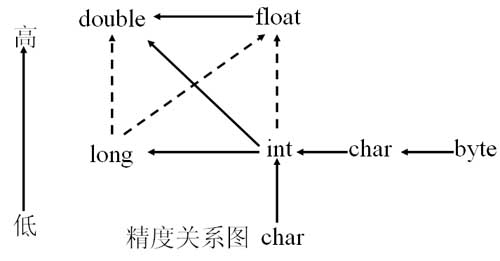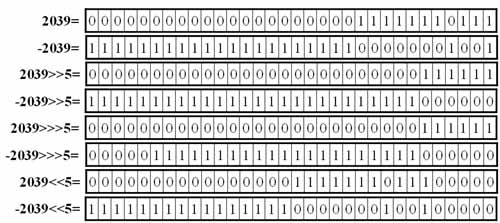# Java中的运算符（操作符）

Java中的运算符（操作符）

程序的基本功能是处理数据，任何编程语言都有自己的运算符。因为有了运算符，程序员才写出表达式，实现各种运算操作，实现各种逻辑要求。

为实现逻辑和运算要求，编程语言设置了各种不同的运算符，且有优先级顺序，所以有的初学者使用复杂表达式的时候搞不清楚。这里详细介绍一下Java中的运算符。

Java运算符很多，下面按优先顺序列出了各种运算符。

 优先级 运算符分类 结合顺序 运算符 由 高 到 低 分隔符 左结合 .    []     ( )     ;      , 一元运算符 右结合 !  ++     --     -   ~ 算术运算符 移位运算符 左结合 *     /      %    +     -      <<   >>   >>> 关系运算符 左结合 <     >     <=   >=   instanceof(Java 特有)   = =  != 逻辑运算符 左结合 ! &&  ||  ~  &  |  ^ 三目运算符 右结合 布尔表达式?表达式1:表达式2 赋值运算符 右结合 =  *=     /=  %=   +=   -=    <<= >>= >>>=  &=  *=  |=

因操作数是一个，故称为一元运算符。

 运算符 含义 例子 - 改变数值的符号，取反 -x（-1*x） ~ 逐位取反，属于位运算符 ~x ++ 自加1 x++ -- 自减1 x--

++x 因为++在前，所以先加后用。
x++ 因为++在后，所以先用后加。

int a=10;
int b=10;
int sum=a+ ++b;
System.out.println("a="+a+",b="+b+",sum="+sum);

int a=10;
int b=10;
int sum=a+++b;
System.out.println("a="+a+",b="+b+",sum="+sum);

n=10;
m=~n;

所谓算术运算符，就是数学中的加、减、乘、除等运算。因算术运算符是运算两个操作符，故又称为二元运算符。

 运算符 含义 例子 + 加法运算 x+y - 减法运算 x-y * 乘法运算 x*y / 除法运算 x/y % 取模运算（求余运算） x%y

这些操作可以对不同类型的数字进行混合运算，为了保证操作的精度，系统在运算过程中会做相应的转化。数字精度的问题，我们在这里不再讨论。下图中展示了运算过程中，数据自动向上造型的原则。注：1、实线箭头表示没有信息丢失的转换，也就是安全性的转换，虚线的箭头表示有精度损失的转化，也就是不安全的。
2、当两个操作数类型不相同时，操作数在运算前会子松向上造型成相同的类型，再进行运算。

5+5.0=10.0
5-5.0=0.0
5*5.0=25.0
22/5=4
22%5=2
22/5.0=4.4
22%5.0=2.0

移位运算符操作的对象就是二进制的位，可以单独用移位运算符来处理int型整数。

 运算符 含义 例子 << 左移运算符，将运算符左边的对象向左移动运算符右边指定的位数（在低位补0） x<<3 >> "有符号"右移运算 符，将运算符左边的对象向右移动运算符右边指定的位数。使用符号扩展机制，也就是说，如果值为正，则在高位补0，如果值为负，则在高位补1. x>>3 >>> "无符号"右移运算 符，将运算符左边的对象向右移动运算符右边指定的位数。采用0扩展机制，也就是说，无论值的正负，都在高位补0. x>>>3运行结果：

1100010011001
11111111111111111110011101100111
11000100
11111111111111111111111100111011
11000100
111111111111111111100111011
110001001100100000
11111111111111001110110011100000

从计算速度上讲，移位运算要比算术运算快。
如果x是负数，那么x>>>3没有什么算术意义，只有逻辑意义。

Java具有完备的关系运算符，这些关系运算符同数学中的关系运算符是一致的。具体说明如下：

 运算符 含义 例子 < 小于 x 大于 x>y <= 小于等于 x<=y >= 大于等于 x>=y == 等于 x==y != 不等于 x!=y

instanceof操作符用于判断一个引用类型所引用的对象是否是一个类的实例。操作符左边的操作元是一个引用类型，右边的操作元是一个类名或者接口，形式如下：

obj instanceof ClassName      或者    obj instanceof InterfaceName

 A !A true false false true

 A B A&&B false false false true false false false true false true true true

 A B A||B false false false true false true false true true true true true

if(1==1 && 1==2 && 1==3){  }

“短路”现象在多重判断和逻辑处理中非常有用。我们经常这样使用：

 A ~A 1 0 0 1

 A B A&B 1 1 1 1 0 0 0 1 0 0 0 0

 A B A | B 1 1 1 1 0 1 0 1 1 0 0 0

 A B A&B 1 1 0 1 0 1 0 1 1 0 0 0

15&2=2
15|2=15
15^2=13

 a 1 1 1 1 15 b 0 0 1 0 2 a&b 0 0 1 0 2 a|b 1 1 1 1 15 a^b 1 1 0 1 13

三目运算符是一个特殊的运算符，它的语法形式如下：

int sum=90;
String str=sum<100 ? "失败" : "成功";

String str=null;
if(num<100){
str="失败";
}else{
str="成功";
}

 运算符 例子 含义 += x+=y x=x+y -= x-=y x=x-y *= x*=y x=x*y /= x/=y x=x/y %= x%=y x=x%y >>= x>>=y x=x>>y >>>= a>>>=y x=x>>>y <<= a<<=y x=x<

04-272万+05-119546
09-091万+
04-121908
02-2311
04-26543
07-118993
08-192282
08-24147
©️2020 CSDN 皮肤主题: 大白 设计师:CSDN官方博客